﻿ Possibility of Cosmic Time Going Back in Some Dark Energy Model Universe

### Possibility of Cosmic Time Going Back in Some Dark Energy Model Universe

Koijam Manihar Singh, Kangujam Priyokumar Singh, Mukunda Dewri

International Journal of Physics

## Possibility of Cosmic Time Going Back in Some Dark Energy Model Universe

Koijam Manihar Singh1, Kangujam Priyokumar Singh2, Mukunda Dewri2,1Department Mathematics, National Institute of Technology, Manipur, Imphal, India

2Department Mathematical Sciences, Bodoland University, Kokrajhar, BTC, Assam, India

### Abstract

In the course of investigating a universe dominated by phantom dark energy it is found that the idea of negative cosmic time, or in other words, reversing the present arrow of time is valid in such a universe from big rip singularity, thus giving us a realistic universe.

• Koijam Manihar Singh, Kangujam Priyokumar Singh, Mukunda Dewri. Possibility of Cosmic Time Going Back in Some Dark Energy Model Universe. International Journal of Physics. Vol. 3, No. 5, 2015, pp 213-215. http://pubs.sciepub.com/ijp/3/5/3
• Singh, Koijam Manihar, Kangujam Priyokumar Singh, and Mukunda Dewri. "Possibility of Cosmic Time Going Back in Some Dark Energy Model Universe." International Journal of Physics 3.5 (2015): 213-215.
• Singh, K. M. , Singh, K. P. , & Dewri, M. (2015). Possibility of Cosmic Time Going Back in Some Dark Energy Model Universe. International Journal of Physics, 3(5), 213-215.
• Singh, Koijam Manihar, Kangujam Priyokumar Singh, and Mukunda Dewri. "Possibility of Cosmic Time Going Back in Some Dark Energy Model Universe." International Journal of Physics 3, no. 5 (2015): 213-215.

 Import into BibTeX Import into EndNote Import into RefMan Import into RefWorks

### 1. Introduction

It is generally assumed that the present accelerated expansion of the universe is mostly due to the presence of an exactly unknown matter, which is generally given the name of dark energy. Many researchers studied possible different forms of dark energy. Among them phantom energy is also one interesting form of dark energy. Different model universes containing phantom dark energy were studied, among others, by [1-28] and Mahanta et al. , also studied some kinds of dark energy model universes. Also recently Martin  studied about the time arrow in a finite universe.

Here in the study of a universe containing such type of dark energy we get a stimulating result in relation to big rip singularity where such a situation can be saved. According to some researchers there is no possibility of big rip singularity in our universe and rather there is possibility of this universe to be a cyclic one. Very interestingly here in this problem, we find that the big rip singularity in such a universe can be saved if we take the cosmic time to go backward; or in other word, the reversal of the present arrow of time is possible in such an epoch.

### 2. Solutions of a New Model Universe

In a homogeneous scalar field the energy density and pressure are given by(1)(2)

Thus in a phantom energy dominated universe where the phantom scalar is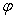, we have(3)

where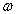is the equation of state parameter.

Since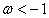in the case of phantom energy, from (3) we get(4)

which gives(5)

Thus, showing that there is negative kinetic energy in the case of phantom energy universe. Hence action for phantom may be taken in the form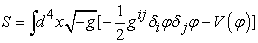(6)

Therefore, in the case of the phantom the energy conservation equation takes the form(7)

With(8)

Now from (7), we get(9)

where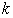being an integration constant.

On the other hand for a spatially flat Friedman Robertson Walker universe metric is(10)

From its field equations, we get the relations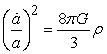(11)

And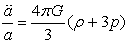(12)

Now from (9) and (11), we have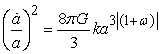(13)

which gives(14)

where(15)andis the present time.

Here we see that, asand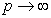. Thus it predicts big rip singularly at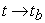.

Now if we introduce the concept of negative time, that means the cosmic time going backward, we have, as,(16)

Thus, in this case there will be not be the catastrophic situation where celestial bodies are expected to get smashed to tiny particles.

For this case we haveThus giving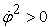(17)

Thereby we have positive kinetic energy when our new time is going back opposite to our present time that is in the negative direction of our usual time thus showing the possibility of reversal of time.

### 3. Conclusions

Here we study a universe dominated by phantom type dark energy and find that a big rip singularity can be avoided if we consider the time to run in the opposite direction of the present cosmic time. In this way we see that there is possibility of reversing the arrow of time at some practical event of time and place. Since there is no big rip singularity in a realistic universe (so far the present scientific knowledge goes). An interesting conclusion that can be made from the result of our present research is that, if there is universe dominated by phantom type of dark energy then in this universe, in some particular event, the phenomenon of ageing can be stopped for some particular period of time.

In the cyclic model of the universe  the transition from the “big crunch” to the “big bang” occurs as the scalar fieldand then rebounds towards positive. Motivated by string theory, it is proposed that some small fraction of the- field kinetic energy is converted to matter and radiation. And the matching rule amounts to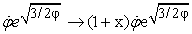(18)

whereis a parameter measuring the efficiency of production of radiation at the bounce. Here, if the concept of negative time is introduced then the expressionhas a physical meaning, otherwise imaginary; similarly in the case with the matching rule defined above which is physically possible when a negative arrow of time is introduced.

We also see that, as the universe contracts towards the “big crunch”, the scale factor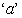tends to zero, the scalar field tends to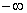and the scalar potential becomes negligible . The universe becomes, dominated by the scalar field kinetic energy density since it scales as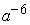, whereas matter and radiation densities scale as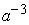and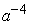respectively. As scalar kinetic energy domination occurs, the scale factor ‘a ’begins to scale as, and the background scale field diverges logarithmically in time.The energy density and Ricci scalar diverge asso that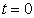is a “big crunch” singularity. The relations involved here imply that there exists the negative of time, as otherwise many a thing pertaining to this universe become imaginary which ultimately implies that the universe will be imaginary, which is impossible. Thus for the universe to be realistic one, as it is now, we have to accept the concept the negative time or reversed of our present cosmic time.

Again, if we consider the bounce cosmology in the universe it can be asserted the bounce takes place atso that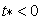represents the contracting phase whereascan be taken to represent the expanding phase . The bounce time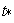is very small; and the universe has a collapsing era for, and there after an expanding era begins. Thus, we see that the concept of negative time comes into the picture when we want to describe the time of an event in the contracting phase just before another cycle begins with an expanding era. The negative time is with respect to the frame of reference of time in the expanding phase of a new cycle of the universe or with respect to the present arrow of time. Further research in the direction is being carried on and the results will be reported somewhere.

### References

  Caldwell, R. R., “A Phantom Menace? Cosmological consequences of a dark energy component with super-negative equation of state”, Phys. Lett. B, 545, 23, September 2002.In article View Article  Hao, J.G.; Li, X.Z., “Attractor solution of phantom field”, Phys. Rev. D, 67, 07303, May 2003.In article View Article  Gibbons, G. W., “Thoughts on Tachyon Cosmology”, Class. Quant. Grav., 20, S321, May 2003.In article View Article  Nojiri, S.; Odintsov, S. D., “Quantum de-Sitter cosmology and phantom matter”, Phys. Lett. B, 562, 147, May 2003.In article View Article  Onemli, V. K.; Woodard, R.P., “Super-Acceleration from Massless, Minimally Coupled phi**4”, Class. Quant. Grav., 19, 4607, August 2002.In article View Article  Carroll, S. M.; Hoffman M.; Trodden, M., “Can the dark energy equation-of-state parameter w be less than -1?”, Phys. Rev. D, 68, 023509, July 2003.In article View Article  Singh P., Sami M.; Dadhich N., “Cosmological Dynamics of Phantom Field”, Phys. Rev. D, 68, 023522, July 2003.In article View Article  Frampton, P H., “Stability Issues for w < -1 Dark Energy”, Mod. Phys. Lett. A , 19, 801, April 2004, hep-th/0302007.In article View Article  Gonzalez-Diaz, P. F., “You need not be afraid of phantom energy”, Phys. Rev. D, 68, 021303, July 2003.In article View Article  Dabrowski, M. P.; Stachowiak, T.; Szydlowski, M., “Phantom cosmologies”, Phys. Rev. D, 68, 103519, Noveber 2003.In article  Elizalde E.; Nojiri S.; Odintsov, S. D., “Late-time cosmology in (phantom) scalar-tensor theory: dark energy and the cosmic speed-up”, Phys. Rev. D, 2004, 70, 043539, August 2004.In article  Nojiri, S.; Odintsov S. D.; Tsujikawa S., “Properties of singularities in (phantom) dark energy universe”, Phys. Rev. D, 71, 063004, March 2005.In article View Article  Liu, D. J.; Li X. Z., “Cosmic Microwave Background Radiation Constraints on a Modified Chaplygin Gas Model”, Chin. Phys. Lett., 22, 1600, March 2005.In article  Briscese F.; Elizalde E.; Nojiri S.; Odintsov S.D., “Phantom scalar dark energy as modified gravity: understanding the origin of the Big Rip singularity”, Phys. Lett. B, 646, 105, January 2007.In article View Article  Bronnikov, K. A.; Fabris, J. C.; Goncalves, S. V. B., “Different faces of the phantom”, Journ. Phys. A, 40, 6835, June 2007, gr-qc/0611038.In article View Article  Bronnikov, K. A.; Starobinsky, A. A., “No realistic wormholes from ghost-free scalar-tensor phantom dark energy”, J E T P Letters, 85, 1, 1-5, March 2007.In article View Article  Manihar, K.; Priyokumar, K., “Cosmological Model Universe Consisting of Two Forms of Dark Energy”, Int.J. Theo. Phys., 53, 4360, May 2004.In article  Adhav, K.S.; Dawande, M.V.; Thakare, R.S.; Raut, R.B., “Bianchi Type-III Magnetized Wet Dark Fluid Cosmological Model in General Relativity”, Int.J. Theo. Phys., 50, 339, October 2010.In article View Article  Ray, F.; Rahaman, F.; Mukhopadhyay, U.; Sarkar, R., “Variable Equation of State for Generalized Dark Energy Model”, Int.J. Theo. Phys., 50, 2687, September 2011.In article View Article  Kumar, S.; Singh, C.P., “Anisotropic dark energy models with constant deceleration parameter”, Gen. Relativ. Gravit., 43, 1427, May 2011.In article View Article  Rao, V. U. M.; Sreedevi, K. G.; Neelima, D., “A dark energy model in a scalar tensor theory of gravitation”, Astrophy. Space Sc., 337, 499, January 2012.In article View Article  Reddy, D. R. K.; Kumar, R. S.; Kumar, T. V. P., “Bianchi type-III Dark Energy Model in f(R,T), Gravity”, Int.J. Theo. Phys., 52, 239-245, January 2013.In article View Article  Samanta, G.C., “Universe Filled with Dark Energy (DE) from a Wet Dark Fluid (WDF) in f(R,T) Gravity”, Int.J. Theo. Phys., 52, 2303, July 2013.In article View Article  Pradhan, A., “Accelerating dark energy models with anisotropic fluid in Bianchi type VI0 space-time”, Res. Astron. Astrophys., 13(2), 139, 2013.In article View Article  Sadeghi, J.; Khurshudyan, M.; Movsisyan, A.; Faraani, H., “Interacting Ghost Dark Energy Models in the Higher Dimensional Cosmology”, January 2014, arXiv:1401.6649v1[gr-qc].In article  Sahoo, P.K.; Mishra, B., “Kaluza-Klein dark energy model in the form of wet dark fluid in f(R, T) gravity”, Can. J. Phys., 92, 1062, July 2014.In article View Article  Sahoo, P. K.; Mishra, B., “Axially symmetric cosmological model with anisotropic dark energy”, Eur. Phys. J. plus, 129,196, September 2014.In article  Samanta, G.C.; Jaiswal, S.; Biswal, S.K., “Universe described by dark energy in the form of wet dark fluid (WDF) in higher-dimensional space-time”, Eur. Phys. J. plus, 129,48, March 2014.In article  Mahanta, K. L.; Biswal, A. K.; Sahoo, P.K., “Bianchi type-III dark energy models with constant deceleration parameter in self-creation cosmology”, Can. J. Phys., 92(4),295, October 2013.In article View Article  Martin, T., “Time’s Arrow in a Finite Universe”, Int. J. Astron. and Astrophy., 5(2), 70 , June 2015.In article View Article  Steinhardt, P. J.; Turok, N., “A Cyclic Model of the Universe”, Science, 296, 1436, May 2002.In article View Article  PubMed  Steinhardt, P.J.; Turok, N., “Cosmic Evolution in a Cyclic Universe”, Phys. Rev. D, 65, 126003, May 2002, arXiv:hep-th/0111098v2.In article View Article  Brevic, I.; Timoshkin, A.V., “Inhomogeneous Dark Fluid and Dark Matter Leading to a Bounce Cosmology”, March 2015 arXiv:1503.02916v1,.In article